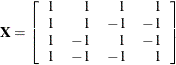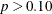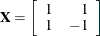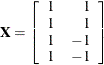### POPULATION Statement

POPULATION variables ;

The POPULATION statement specifies that populations are to be based only on cross-classifications of the specified variables. If you do not specify the POPULATION statement, then populations are based only on cross-classifications of the independent variables in the MODEL statement.

The POPULATION statement has two major uses:

• When you enter the design matrix directly, there are no independent variables in the MODEL statement; therefore, the POPULATION statement is the only way to produce more than one population.

• When you fit a reduced model, the POPULATION statement might be necessary if you want to form the same number of populations as there are for the saturated model.

To illustrate the first use, suppose you specify the following statements:

data one;
input A $B$ wt @@;
datalines;
yes yes 23   yes no 31   no yes 47   no no 50
;

proc catmod;
weight wt;
population B;
model A=(1 0,
1 1);
run;


Since the dependent variable A has two levels, there is one response function per population. Since the variable B has two levels, there are two populations. The MODEL statement is valid since the number of rows in the design matrix (2) is the same as the total number of response functions. If the POPULATION statement is omitted, there would be only one population and one response function, and the MODEL statement would be invalid.

To illustrate the second use, suppose you specify the following statements:

data two;
input A $B$ Y wt @@;
datalines;
yes  yes  1  23       yes  yes  2  63
yes  no   1  31       yes  no   2  70
no   yes  1  47       no   yes  2  80
no   no   1  50       no   no   2  84
;

proc catmod;
weight wt;
model Y=A B A*B / wls;
run;


These statements form four populations and produce the following design matrix and analysis of variance table:Source

DF

Chi-Square

Pr > ChiSq

Intercept

1

48.10

<.0001

A

1

3.47

0.0625

B

1

0.25

0.6186

A*B

1

0.19

0.6638

Residual

0

Since the B and A*B effects are nonsignificant (), fit the reduced model that contains only the A effect:

proc catmod;
weight wt;
model Y=A / wls;
run;


Now only two populations are formed, and the design matrix and the analysis of variance table are as follows:Source

DF

Chi-Square

Pr > ChiSq

Intercept

1

47.94

<.0001

A

1

3.33

0.0678

Residual

0

However, you can form four populations by adding the POPULATION statement to the analysis:

proc catmod;
weight wt;
population A B;
model Y=A / wls;
run;


The design matrix and the analysis of variance table resulting from these statements are as follows:Source

DF

Chi-Square

Pr > ChiSq

Intercept

1

47.76

<.0001

A

1

3.30

0.0694

Residual

2

0.35

0.8374

The advantage of the latter analysis is that it retains four populations for the reduced model, thereby creating a built-in goodness-of-fit test: the residual chi-square. Such a test is important because the cumulative (or joint) effect of deleting two or more effects from the model can be significant, even if the individual effects are not.

The resulting differences between the two analyses are due to the fact that the latter analysis uses pure weighted least squares estimates with respect to the four populations that are actually sampled. The former analysis pools populations and therefore uses parameter estimates that can be regarded as weighted least squares estimates of maximum likelihood predicted cell frequencies. In any case, the estimation methods are asymptotically equivalent; therefore, the results are very similar. If you specify the ML option (instead of the WLS option) in the preceding MODEL statements, then the parameter estimates are identical for the two analyses.

Caution: If your model has different covariate profiles within any population, then the first profile is used in the analysis.